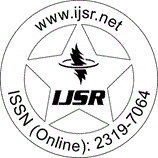International Journal of Science and Research (IJSR)
Call for Papers | Fully Refereed | Open Access | Double Blind Peer Reviewed

Research Paper | Mathematics | Mongolia | Volume 7 Issue 7, July 2018

# The Numerical Range for z1V+a2V*

Abstract: Let V denote the classical Volterra operator on L^2 [0, 1] and let z_1, z_2 be an arbitrary complex numbers. We investigated the numerical range and the numerical radius of z_1V+z_2V^{*}. In particular, we calculated the numerical range and numerical radius of V without using the known results.

Keywords: Volterra operator, numerical range, numerical radius

Edition: Volume 7 Issue 7, July 2018,

Pages: 728 - 730